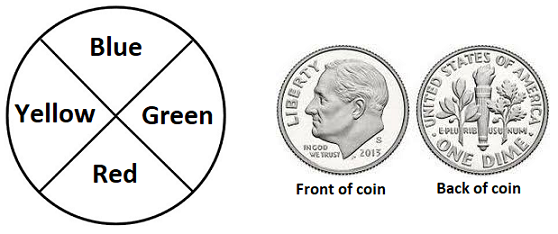# Probability

## Objective

List the sample space for compound events using organized lists, tables, or tree diagrams.

## Common Core Standards

### Core Standards

?

• 7.SP.C.8.B — Represent sample spaces for compound events using methods such as organized lists, tables and tree diagrams. For an event described in everyday language (e.g., "rolling double sixes"), identify the outcomes in the sample space which compose the event.

## Criteria for Success

?

1. Understand the difference in sample spaces between a simple event and a compound event.
2. Represent sample spaces of compound events using organization tools including lists, tables, and tree diagrams.
3. Understand the probability of a compound event, similar to a simple event, is represented as a fraction, where the numerator is the number of desired events and the denominator is the number of total events in the sample space.
4. Find probabilities of compound events using the sample space.

## Tips for Teachers

?

Following the introduction to compound events in Lesson 6, in this lesson students learn how to organize the sample space for compound events. Within each organizational tool, there is a structure embedded that ensures each possible outcome is accounted for (MP.7). Ensure students see and can utilize this structure as they create their own organized spaces.

#### Fishtank Plus

• Problem Set
• Student Handout Editor
• Vocabulary Package

## Anchor Problems

?

### Problem 1

An experiment involves a spinner and a fair coin, shown below. The spinner will be spun one time, and the coin will be flipped one time.What are all of the possible outcomes for this experiment? Organize your information in a list, table, or tree diagram.

### Problem 2

Imagine a game in which two fair four-sided (tetrahedral) dice are rolled simultaneously. These dice are in the shape of a pyramid, and when a die is rolled, the outcome is determined by the side that lands face down. Suppose that for these two dice, the possible values (corresponding to the four sides of the die) that can be obtained from each die are as follows:

Die #1: 1, 2, 3, or 4

Die #2: 2, 4, 6, or 8

a.   A certain game determines the movement of players' game pieces based on the SUM of the numbers on the face down sides when two dice are rolled.

1. Using an organized list, table, or tree diagram, develop a list of all 16 possible outcomes.
2. From your work in part (i), determine the 10 distinct sums that are possible and calculate the probability of obtaining each sum.

What is the probability of obtaining a sum of 5?

What is the probability of obtaining a sum that is more than 5?

What is the probability of obtaining a sum that is at most 5?

What is the probability of obtaining a sum that is at least 5?

What is the probability of obtaining a sum that is no less than 5?

b.  Now consider the case where the DIFFERENCE in the numbers on the face down sides when two dice are rolled is important to the game. Unless the two die values are the same (in which case the difference is 0), the difference for purposes of this game will always be computed as the larger number value rolled minus the smaller number value rolled. In this way, the difference value for any roll of the two dice will always be positive or 0.

1. Using an organized list, table, or tree diagram, develop a list of all 16 possible outcomes.
2. From your work in part (i), determine the eight distinct differences that are possible and calculate the probability of obtaining each difference.

What is the probability of obtaining a difference of 5?

What is the probability of obtaining a difference that is more than 5?

What is the probability of obtaining a difference that is less than or equal to 5?

#### References

Illustrative Mathematics Tetrahedral Dice

Tetrahedral Dice, accessed on June 14, 2017, 2:44 p.m., is licensed by Illustrative Mathematics under either the CC BY 4.0 or CC BY-NC-SA 4.0. For further information, contact Illustrative Mathematics.

### Problem 3

At an ice cream store, a customer can make a sundae that includes ice cream, one candy topping, and one liquid topping. The options are listed below.

 Ice cream flavors Candy toppings Liquid toppings Chocolate Butterfinger Hot fudge Mint chip M&M's Marshmallow Vanilla Gummy bears Reese's
1. How many different sundae combinations are possible?
2. Use a list, table, or tree diagram to show all possible outcomes.
3. How many different sundaes include mint chip ice cream and hot fudge topping?
4. Assuming each sundae combination is equally likely, what is the probability that the store’s next customer orders a sundae with vanilla ice cream and gummy bears?

## Problem Set

?The following resources include problems and activities aligned to the objective of the lesson that can be used to create your own problem set.

?

An experiment involves flipping a fair coin and rolling a fair six-sided die.

a.   List all possible outcomes of the experiment. Use an organized list, table, or tree diagram.

b.   What is the probability of getting a head and an even number?

c.   What is the probability of getting a tail and the number 4?

d.   What is the probability of getting a 5?